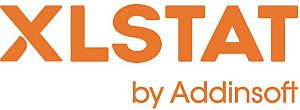# XLSTAT - Discriminant Analysis (DA)

## What is Discriminant Analysis?

Discriminant Analysis (DA) is an old method (Fisher, 1936) which in its classic form has changed little in the past twenty years. This method, which is both explanatory and predictive, can be used to:

• Check on a two- or three-dimensional chart if the groups to which observations belong are distinct,
• Show the properties of the groups using explanatory variables,
• Predict which group an observation will belong to

is Discriminant Analysis may be used in numerous applications, for example in ecology and the prediction of financial risks (credit scoring).

## Model for Discriminant Analysis: Linear or quadratic model

Two models of Discriminant Analysis are used depending on a basic assumption: if the covariance matrices are assumed to be identical, linear discriminant analysis is used. If, on the contrary, it is assumed that the covariance matrices differ in at least two groups, then the quadratic model is used. The Box test is used to test this hypothesis (the Bartlett approximation enables a Chi2 distribution to be used for the test). We start with linear analysis then, depending on the results from the Box test, carry out quadratic analysis if required.

## Discriminant Analysis and Multicolinearity issues

With linear and still more with quadratic models, we can face problems of variables with a null variance or multicolinearity between variables. XLSTAT has been programmed so as to avoid these problems. The variables responsible for these problems are automatically ignored either for all calculations or, in the case of a quadratic model, for the groups in which the problems arise. Multicolinearity statistics are optionally displayed so that you can identify the variables which are causing problems.

## Discriminant Analysis and variable selection

As for linear and logistic regression, efficient stepwise methods have been proposed. They can, however, only be used when quantitative variables are selected as the input and output tests on the variables assume them to be normally distributed. The stepwise method gives a powerful model which avoids variables which contribute only little to the model.

## Discriminant Analysis results: Classification table, ROC curve and cross-validation

Among the numerous results provided, XLSTAT can display the classification table (also called confusion matrix) used to calculate the percentage of well-classified observations. When only two classes (or categories or modalities) are present in the dependent variable, the ROC curve may also be displayed.

The ROC curve (Receiver Operating Characteristics) displays the performance of a model and enables a comparison to be made with other models. The terms used come from signal detection theory.

The proportion of well-classified positive events is called the sensitivity. The specificity is the proportion of well-classified negative events. If you vary the threshold probability from which an event is to be considered positive, the sensitivity and specificity will also vary. The curve of points (1-specificity, sensitivity) is the ROC curve.

Let's consider a binary dependent variable which indicates, for example, if a customer has responded favorably to a mail shot. In the diagram below, the blue curve corresponds to an ideal case where the n% of people responding favorably corresponds to the n% highest probabilities. The green curve corresponds to a well-discriminating model. The red curve (first bisector) corresponds to what is obtained with a random Bernoulli model with a response probability equal to that observed in the sample studied. A model close to the red curve is therefore inefficient since it is no better than random generation. A model below this curve would be disastrous since it would be less even than random.

The area under the curve (or AUC) is a synthetic index calculated for ROC curves. The AUC corresponds to the probability such that a positive event has a higher probability given to it by the model than a negative event. For an ideal model, AUC=1 and for a random model, AUC = 0.5. A model is usually considered good when the AUC value is greater than 0.7. A well-discriminating model must have an AUC of between 0.87 and 0.9. A model with an AUC greater than 0.9 is excellent.

The results of the model as regards forecasting may be too optimistic: we are effectively trying to check if an observation is well-classified while the observation itself is being used in calculating the model. For this reason, cross-validation was developed: to determine the probability that an observation will belong to the various groups, it is removed from the learning sample, then the model and the forecast are calculated. This operation is repeated for all the observations in the learning sample. The results thus obtained will be more representative of the quality of the model. XLSTAT gives the option of calculating the various statistics associated with each of the observations in cross-validation mode together with the classification table and the ROC curve if there are only two classes.

Lastly, you are advised to validate the model on a validation sample wherever possible. XLSTAT has several options for generating a validation sample automatically.

## Discriminant analysis and logistic regression

Where there are only two classes to predict for the dependent variable, discriminant analysis is very much like logistic regression. Discriminant analysis is useful for studying the covariance structures in detail and for providing a graphic representation. Logistic regression has the advantage of having several possible model templates, and enabling the use of stepwise selection methods including for qualitative explanatory variables. The user will be able to compare the performances of both methods by using the ROC curves.This analysis is available in the XLStat-Basic addin for Microsoft ExcelKovach Computing Services (KCS) was founded in 1993 by Dr. Warren Kovach. The company specializes in the development and marketing of inexpensive and easy-to-use statistical software for scientists, as well as in data analysis consulting.

### Get in Touch

• Email:
sales@kovcomp.com